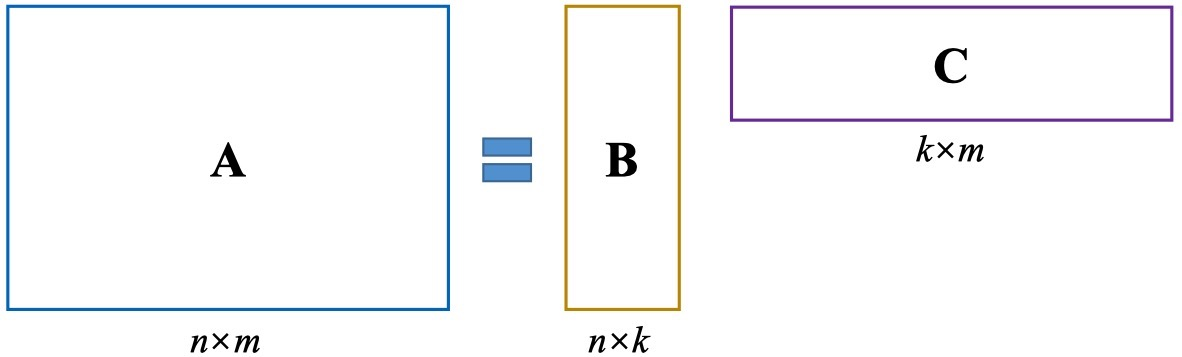## Matrix Decomposition

• PCA: Principle Component Analysis
• SVD: Singular Value Decomposition
• LDA: Linear Discriminant Analysis
• NMF: Non-negative Matrix Factorization
• NMF: with Sparse Constraints
• Linear Sparse Coding

## Overview

$$A_{n \times m} = B_{n \times k}C_{k \times m}$$

• $B$ captures the common features in $A$
• $C$ carries specific characteristics of the original samples
• $n \times m \rightarrow (n+m) \times k$
• $k<<n$• In PCA: $B$ is eigenvectors
• In SVD: $B$ is right (column) eigenvectors
• In LDA: $B$ is discriminant directions
• In NMF: $B$ is local features